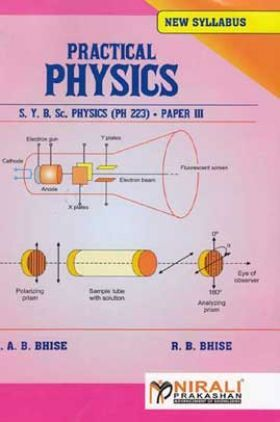# Practical Physics

2888 Views
MRP : ₹95.00
Price : ₹66.50
You will save : ₹28.50 after 30% Discount
Inclusive of all taxes

Save extra with 2 Offers

Get ₹ 50

Instant Cashback on the purchase of ₹ 400 or above

Product Specifications

 Publisher Nirali Prakashan All B.Sc. - Bachelor of Science books by Nirali Prakashan ISBN 9789351644620 Author: Dr. A. B. Bhise, R. B. Bhise Number of Pages 109 Available Available in all digital devicesPractical Physics by Dr. A. B. Bhise, R. B. Bhise
Book Summary:
We are happy to place this book “S.Y.B.Sc. Physics Practical” in the hand of B.Sc. students and teachers of Savitribai Phule Pune University. This book envisages the revised syllabus which is implemented from the academic year 2014-15. It is general impression of physics students that physics practicals are very difficult, hence the experimental matter is prescribed in a simple and lucid manner with the help of neat and clear figures of instruments.

This book gives a brief description of physics experiments which are chosen as per the latest curriculum. The experiments include oscillations, waves, sound, optics, electronics, instrumentation, computer and demonstration. Each experiment is discussed with Aim, Apparatus, Diagram, Procedure, Observation, Observation table, Formulae and Results. The theory of experiment is presented in brief in the principle with necessary formulae and their explanation.

At the end of each experiment necessary Oral Questions, Tables and Graphs are included. We think that the students will find this book most useful while performing the experiments.

Audience of the Book :
This book Useful for B.Sc students.

Section - I

1. Logarithmic decrement (in air and water)

2. Study of coupled oscillators comprising two simple pendulum (mechanical) and determination of coupling coefficient.

3. Determination of frequency of AC mains using sonometer.

4. Directional characteristics of Microphone.

5. Newton’s Rings : Determination of wavelength of monochromatic light source

6. Dispersive power of glass prism

7. Total internal reflection (using a LASER beam and glass prism).

8. Diffraction at the edge of a razor blade.

9. Measurement of coefficient of absorption of sound for different materials (cork, thermocol, mica, paper etc.)

10. Velocity of sound by phase shift method.

11. Optical activity of sugar solution (polarimeter).

12. Double refracting prism.

Section - II

1. Circuit Theorems. (Thevenin’s, Norton’s and Maximum power transfer theorem)

2. Transistor characteristics (CE configuration).

3. Transistor amplifier (single stage)

4. Study of rectifiers (half wave and full wave) with different filters.

5. I-V characteristics of UJT

6. UJT as a relaxation oscillator.

7. Zener as a regulator, line and load regulation.

8. Study of phase shift oscillator (using IC 741)

9. OPAMP as inverting and non-inverting amplifier

10. Study of logic gates (using IC) and verification of De Morgan’s theorems.

11. Use of CRO (AC/DC voltage measurement, frequency measurement).

12. Plotting various trigonometric functions using spreadsheet/any graphic software :sin (, cos (), tan (), ey, ey, log (x), ln (x), xn